# How to Divide in Google Sheets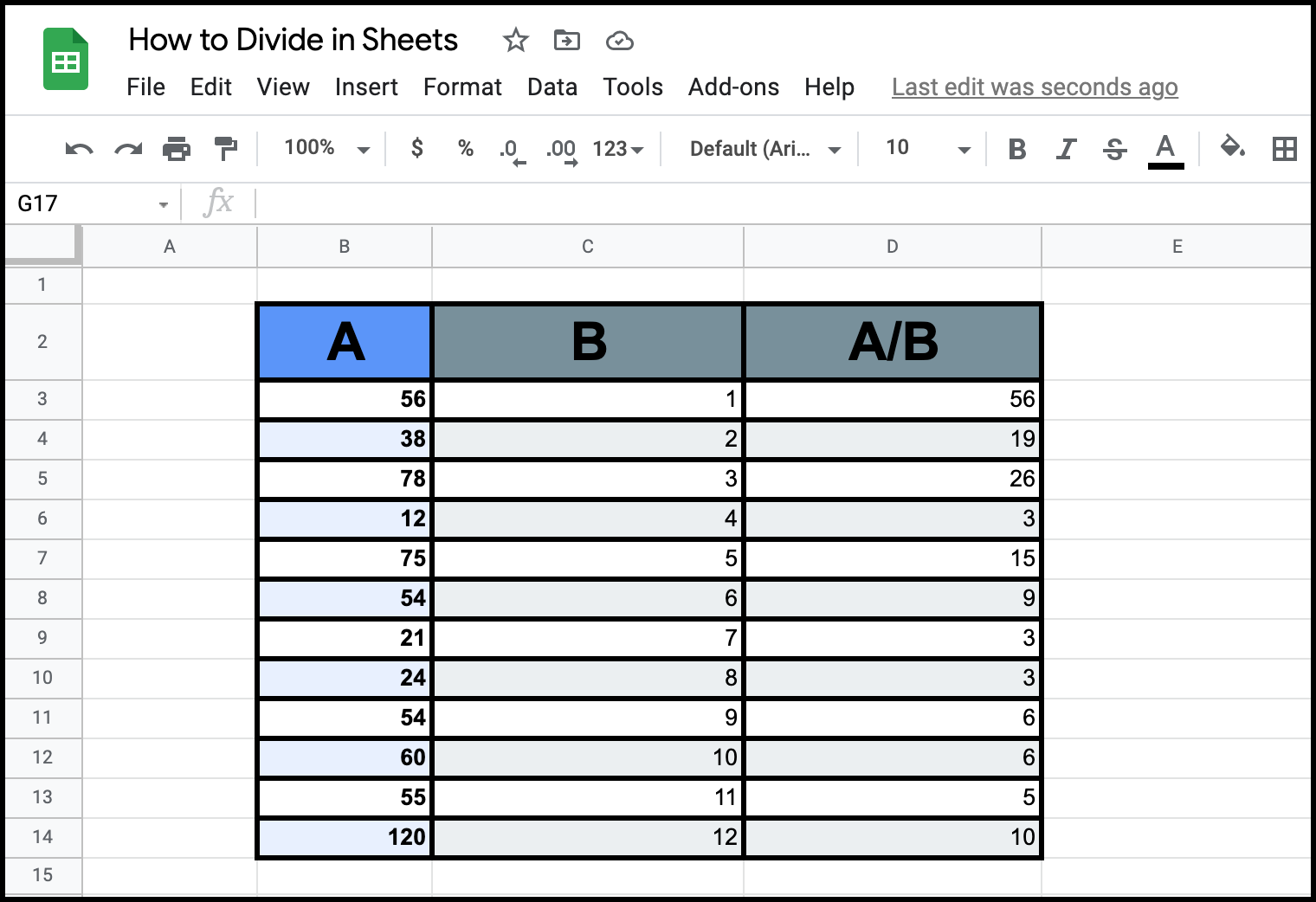In all analytics, everyone will eventually need to know how to divide massive columns of data against each other. Dividing in google sheets is one of the simpler functions you can perform on Google Sheets.

These 3-Steps will show you how to easily divide massive amounts of data.

## Step #1: Select the Cell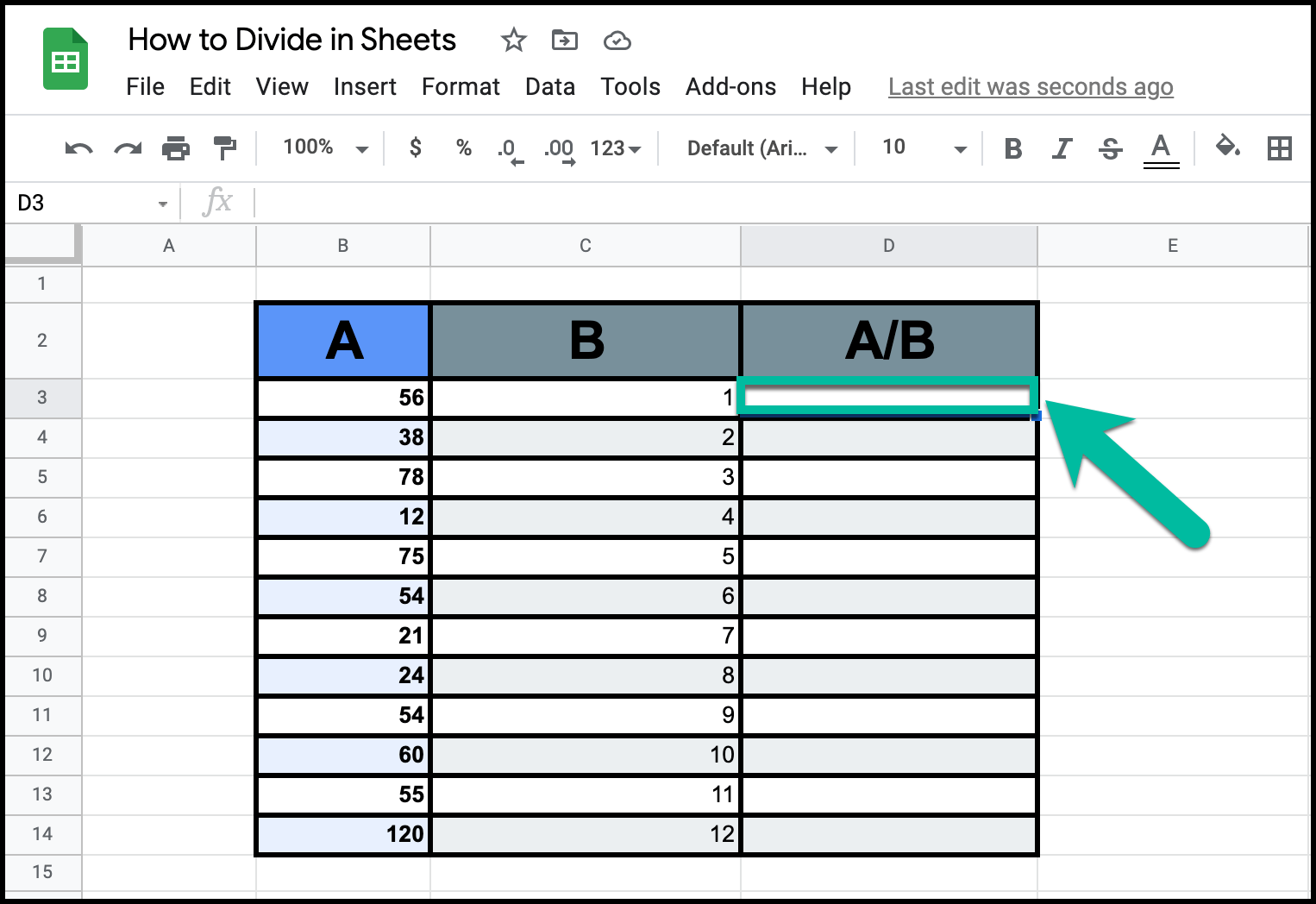First, you need to click the "Cell" where you want the result of the division to appear. You should select the topmost cell of that column, as that will help you fill out the rest of your chart later.

## Step #2: Command Function

There are two functions that you can use to multiply the two cells together:

"=DIVIDE(Cell1,Cell2)" and "=Cell1/Cell2"

### "=DIVIDE(Cell1,Cell2)"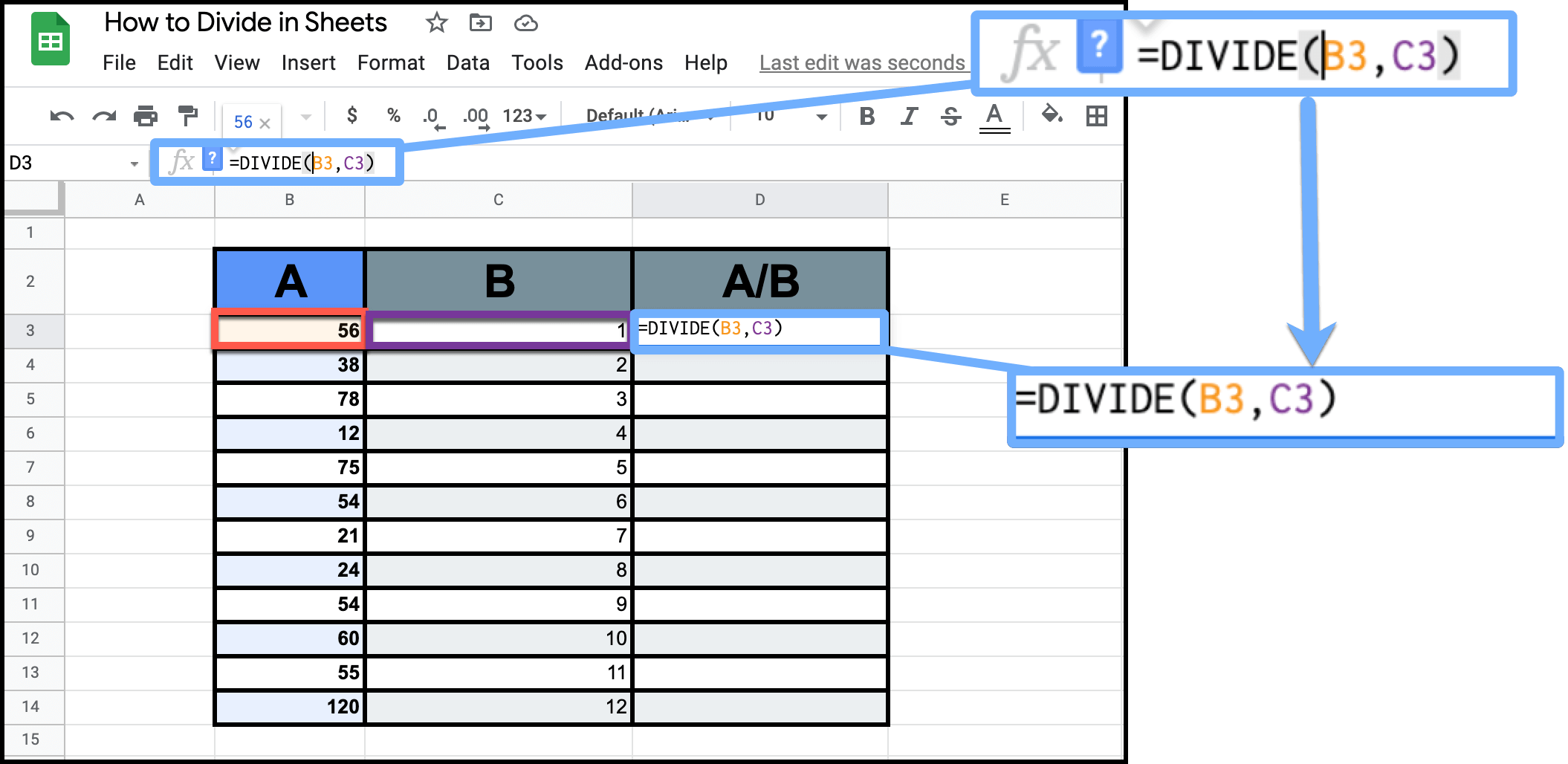Now, simply use the "=DIVIDE()" function, which takes advantage of cell references. Then, you will type in the cell coordinates of the numerator first, then follow that by a comma. (It is really important that you add the comma). Then it uses the same division formula as "x/y".

Next, you will add the cell coordinates of the denominator. From there, simply hit enter and your result should appear in the cell that you selected in Step #1.

## "=Cell1/Cell2"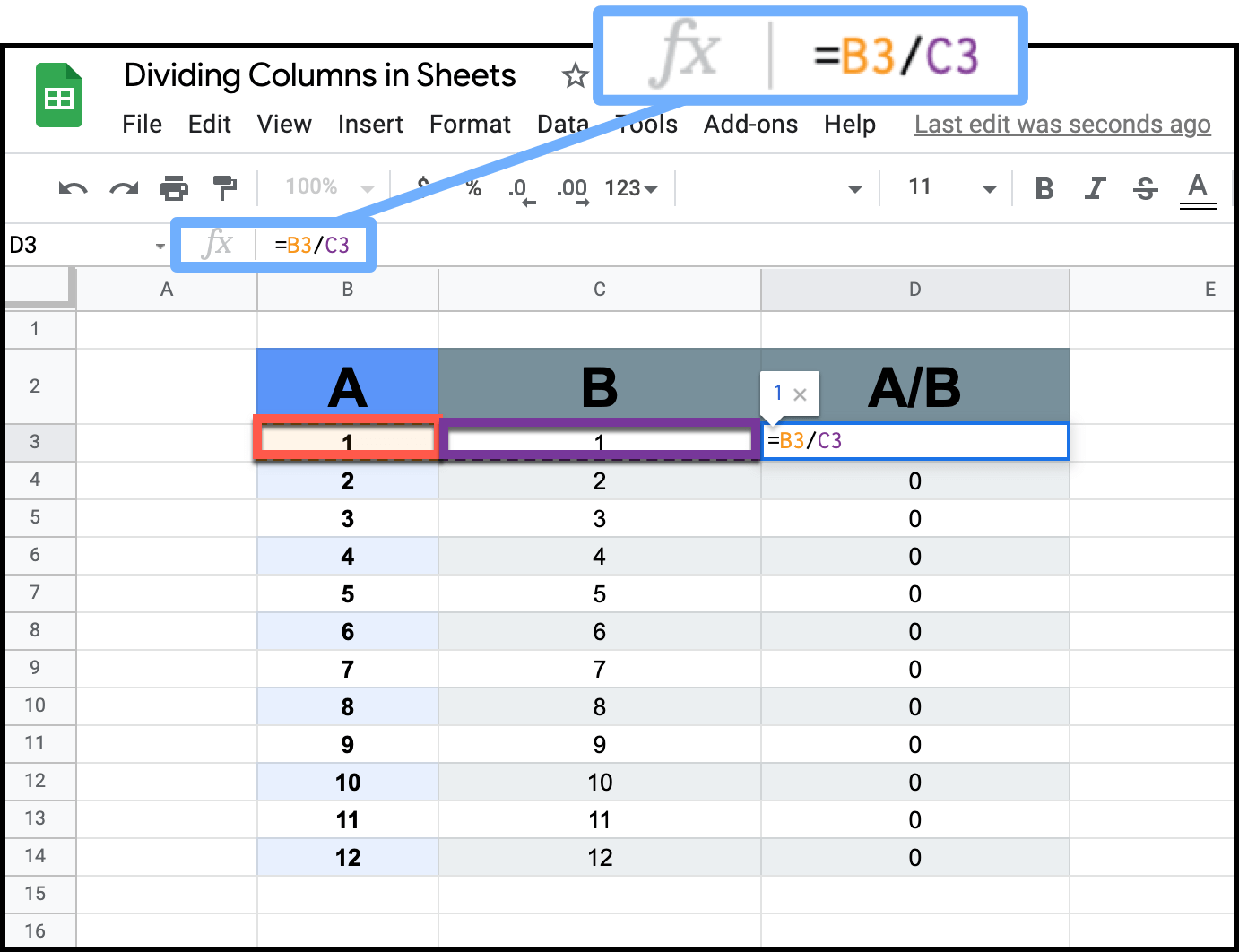Alternatively, in the case of the example shown above, you could divide the cells together by typing "=B3/C3". That will have the same effect as the "=DIVIDE()" function, but it can be used instead of that division formula.

Many people prefer this method because it feels more like you are using a calculator. As such, this technique comes more naturally to people.

## Step #3: Autofill the Column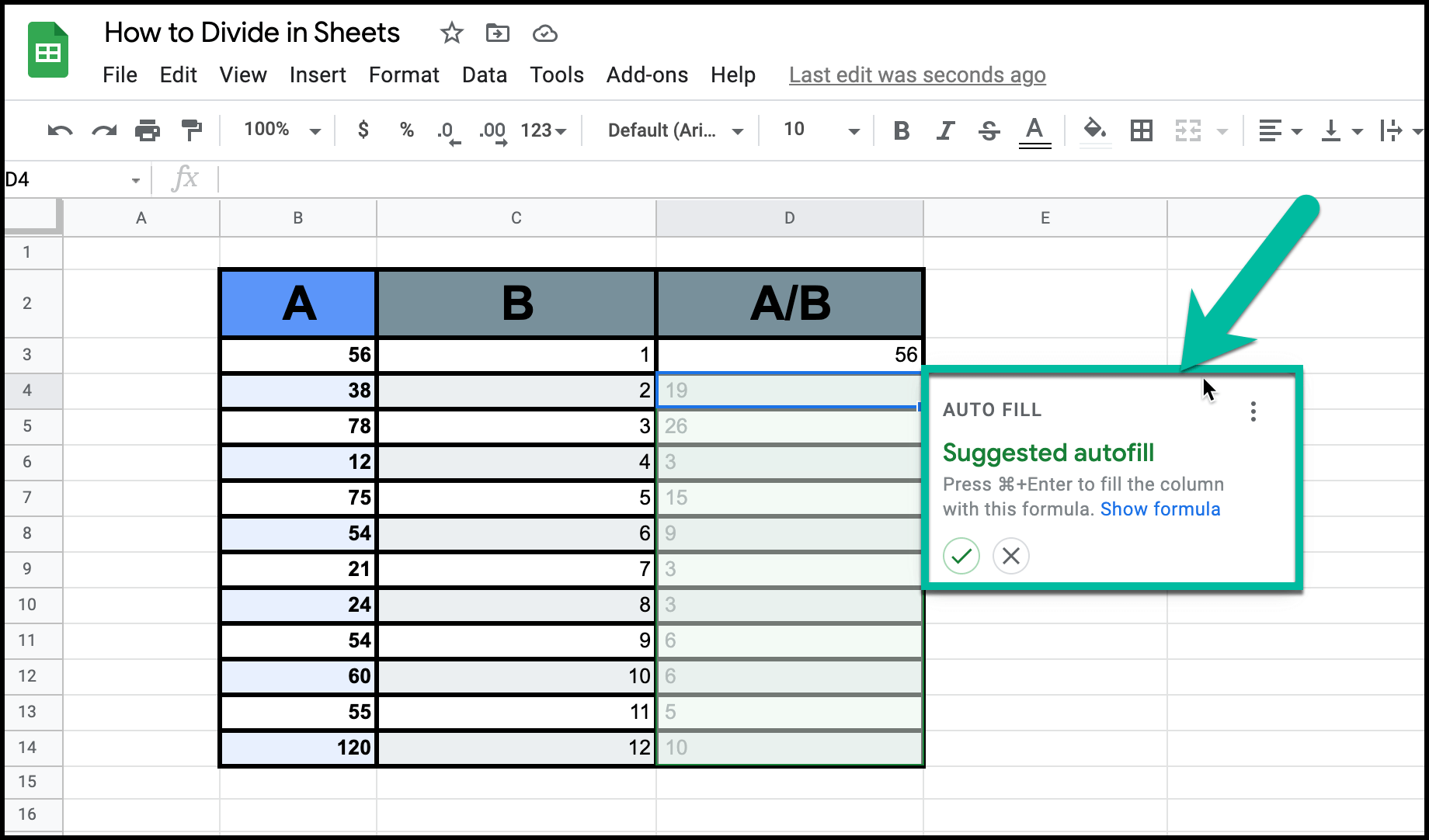Once you enter the value for the first cell, you should see a prompt to autofill the rest of your column in the same way. If that doesn't work, simply click and drag from the bottom corner of the original cell.

The only difference is that the numerator and denominator will change as it descends the column. Using this function allows you to divide entire columns against each other.